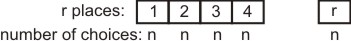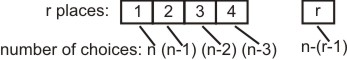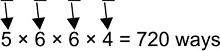#### Total number of four digit odd numbers that can be formed using 0, 1, 2, 3, 5, 7 are Option 1) 216 Option 2) 375 Option 3) 400 Option 4) 720As we learnt in

Number of Permutations with repetition -

The number of ways of filling r places where each place can be filled by any one of  n objects.It is- wherein

WhereNumber of Permutations without repetition -

Arrange n objects taken r at a time equivalent to filling r places from n things.- wherein

WhereWe have 0, 1, 2, 3, 5, 7 and Repetition is allowed.Correct option is 4.

Option 1)

216

This is an incorrect option.

Option 2)

375

This is an incorrect option.

Option 3)

400

This is an incorrect option.

Option 4)

720

This is the correct option.

#### Plabita